Test: Soil Mechanics- 1

# Test: Soil Mechanics- 1

Test Description

## 10 Questions MCQ Test GATE Civil Engineering (CE) 2023 Mock Test Series | Test: Soil Mechanics- 1

Test: Soil Mechanics- 1 for Civil Engineering (CE) 2023 is part of GATE Civil Engineering (CE) 2023 Mock Test Series preparation. The Test: Soil Mechanics- 1 questions and answers have been prepared according to the Civil Engineering (CE) exam syllabus.The Test: Soil Mechanics- 1 MCQs are made for Civil Engineering (CE) 2023 Exam. Find important definitions, questions, notes, meanings, examples, exercises, MCQs and online tests for Test: Soil Mechanics- 1 below.
Solutions of Test: Soil Mechanics- 1 questions in English are available as part of our GATE Civil Engineering (CE) 2023 Mock Test Series for Civil Engineering (CE) & Test: Soil Mechanics- 1 solutions in Hindi for GATE Civil Engineering (CE) 2023 Mock Test Series course. Download more important topics, notes, lectures and mock test series for Civil Engineering (CE) Exam by signing up for free. Attempt Test: Soil Mechanics- 1 | 10 questions in 30 minutes | Mock test for Civil Engineering (CE) preparation | Free important questions MCQ to study GATE Civil Engineering (CE) 2023 Mock Test Series for Civil Engineering (CE) Exam | Download free PDF with solutions
 1 Crore+ students have signed up on EduRev. Have you?
Test: Soil Mechanics- 1 - Question 1

### If the plasticity index of a soil is 45%, then the soil will be:

Detailed Solution for Test: Soil Mechanics- 1 - Question 1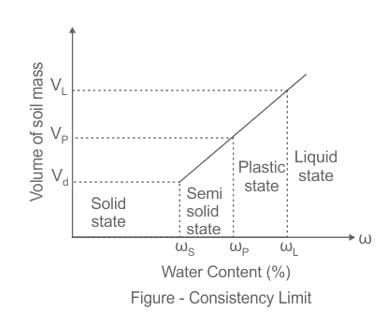Plasticity index (PI) is the range of water content over which the soil remains in the plastic state. Mathematically defined as,

Plasticity Index = Liquid Limit (wL) – Plastic Limit (wp)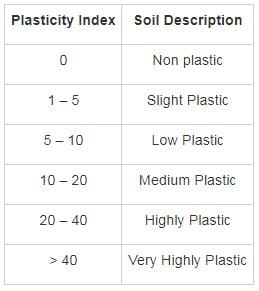Test: Soil Mechanics- 1 - Question 2

### Consider the following statements. The incorrect statement is:

Detailed Solution for Test: Soil Mechanics- 1 - Question 2
1. Water content is the ratio of the mass of water to the mass of solids. It can be greater than 100%.
2. The degree of saturation has ranged from 0% to 100%.
3. The porosity cannot be greater than 100%.
4. The void ratio of soil can be greater than 1.
Test: Soil Mechanics- 1 - Question 3

### The term ‘Loess’ indicates those soils which are 1.  Uniformly graded 2.  Poorly graded 3.  Made up of more than 50% sand size particles 4. Made up of more than 50% of silt particles 5. These are transported by winds Which of the above statements are correct:

Detailed Solution for Test: Soil Mechanics- 1 - Question 3
• Loess is an Aeolian Sediment i.e. transported by winds.
• These contain fine particles like silt and clay (typically in the 20–50 micrometer size range).
• Loess is uniformly graded soil. It is usually homogeneous and highly porous and is traversed by vertical capillaries that permit the sediment to fracture and form vertical bluffs.
Test: Soil Mechanics- 1 - Question 4

Match List – I (Type of soil) with List-II (Mode of transportation and deposition) and select the correct answer using the codes given below the lists: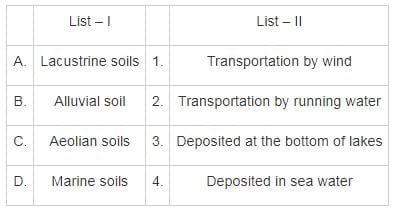Detailed Solution for Test: Soil Mechanics- 1 - Question 4

Lacustrine soil: Soil which is deposited from suspension in fresh still water of the lakes is known as Lacustrine soil. Lacustrine deposits are sedimentary rock formations which formed in the bottom of ancient lakes

Alluvial Soil: Soil which is been deposited from suspension in running water is known as Alluvial soil. It is transported soil formed by physical wheathering.

Aeoline Soil: Soil which is formed due to transportation by wind is known as Aoeline soil. It is also a transported soil.

Marine soil: Soil which is deposited from the suspension in sea water is known as marine soil.

Test: Soil Mechanics- 1 - Question 5

In wet soil mass, air occupies 1/6 of its volume and water occupies 1/3 of its volume. The void ratio of the soil is

Detailed Solution for Test: Soil Mechanics- 1 - Question 5

Voids ratio: It is defined as the ratio of the total volume of voids to the volume of solids in a given soil mass.

Void ratio, e = V/ Vs

e > 0, voids ratio has no upper limit

Vv = air void (Va) + water filled voids (Vw)
Calculation:

Given,

Va = 1/6 of V

Vw = 1/3 of V

Vv = Va + Vw = V/2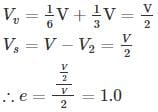Test: Soil Mechanics- 1 - Question 6

The given figure indicate the weights of different pycnometers: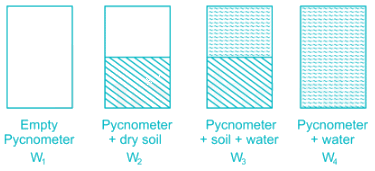The specific gravity of the solids is given by

Detailed Solution for Test: Soil Mechanics- 1 - Question 6

Specific gravity of solids is the ratio of weight of given volume of solids to the weight of equivalent volume of water at 4° C.

Specific gravity is usually reported at 27°C and determined using pycnometer. It is given by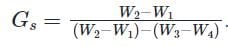W= Mass of empty volume of pycnometer

W2 = Mass of pycnometer + Mass of moist sample

W3 = Mass of pycnometer + soil + water

W4 = Mass of pycnometer full of water

Gs = Specific gravity of soil solids

*Answer can only contain numeric values
Test: Soil Mechanics- 1 - Question 7

The following data are given for soil:

• Porosity: n = 0.4

• The specific gravity of the soil solids: Gs = 2.68

• Moisture content: w = 12%

Determine the mass of water in kg to be added to 10 m3 of soil for full saturation.

Detailed Solution for Test: Soil Mechanics- 1 - Question 7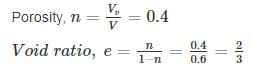Total volume of soil, V = 10 m3

∴ VV = 0.4 × 10 = 4m3

Volume of solids, Vs = V – VV = 10 – 4 = 6 m3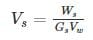Mass of solids, Ws = Vs Gs Yw = 6 × 2.68 × 1000 = 16080 kg

Moisture content = 0.12

So, Mass of water = 0.12 × 16080 = 1929.6 kg

Volume of water = 1929.6/1000 = 1.929 m3

So, phase diagram can be shown as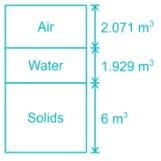For full saturation, the amount of air has to be replaced by water.

So, water to be added = 2.071 × 1000 = 2071 kg

Test: Soil Mechanics- 1 - Question 8

The water content of a soil sample is found to be 35%. If the percentage of air voids present in the soil sample is 5%. Then compute dry unit weight and air content of the soil sample when the void ratio of the soil sample is 0.65.

Take specific gravity of soil solids and unit weight of water to be 2.70 and 10 kN/m3.

Detailed Solution for Test: Soil Mechanics- 1 - Question 8

Dry unit weight is given by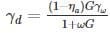ηa = 0.05

G = 2.70

ω = 0.35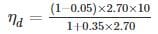ηd = 13.19 kN/m3

ηa = acη

η → porosity

ac → aircontent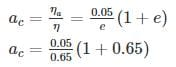ac = 0.1269
ac = 12.70%

*Answer can only contain numeric values
Test: Soil Mechanics- 1 - Question 9

A fully saturated clay has a water content of 40% and unit weight 19 kN/m3. After oven drying the dry density of the soil becomes 18 kN/m3. The shrinkage limit for the soil sample is ______%. (Take γw=γw=10 kN/m3)

Detailed Solution for Test: Soil Mechanics- 1 - Question 9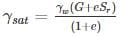And, e sr = wG

⇒ e × 1 = 0.40 G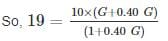⇒ G = 2.97
Shrinkage limit, w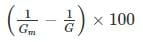Where, Gm = Mass specific gravity at dry state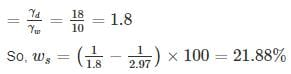*Answer can only contain numeric values
Test: Soil Mechanics- 1 - Question 10

The soil has to be excavated from a borrow-pit which has a density of 1.12 gm/cc with a water content of 12% to fill a land. The soil is compacted to a density of 1.32 gm/cc with water content 20%. Find the volume of soil to be excavated in m3 for 1000 m3 volume of the fill?

Detailed Solution for Test: Soil Mechanics- 1 - Question 10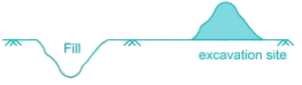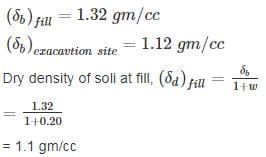Dry density of soil at excavation site,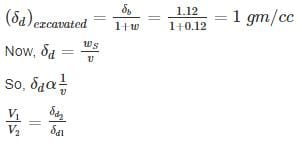∴ Volume of excavated soil =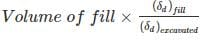= 1000 × 1.1/1
= 1100 m3

## GATE Civil Engineering (CE) 2023 Mock Test Series

27 docs|296 tests
Information about Test: Soil Mechanics- 1 Page
In this test you can find the Exam questions for Test: Soil Mechanics- 1 solved & explained in the simplest way possible. Besides giving Questions and answers for Test: Soil Mechanics- 1, EduRev gives you an ample number of Online tests for practice

## GATE Civil Engineering (CE) 2023 Mock Test Series

27 docs|296 tests(Scan QR code)